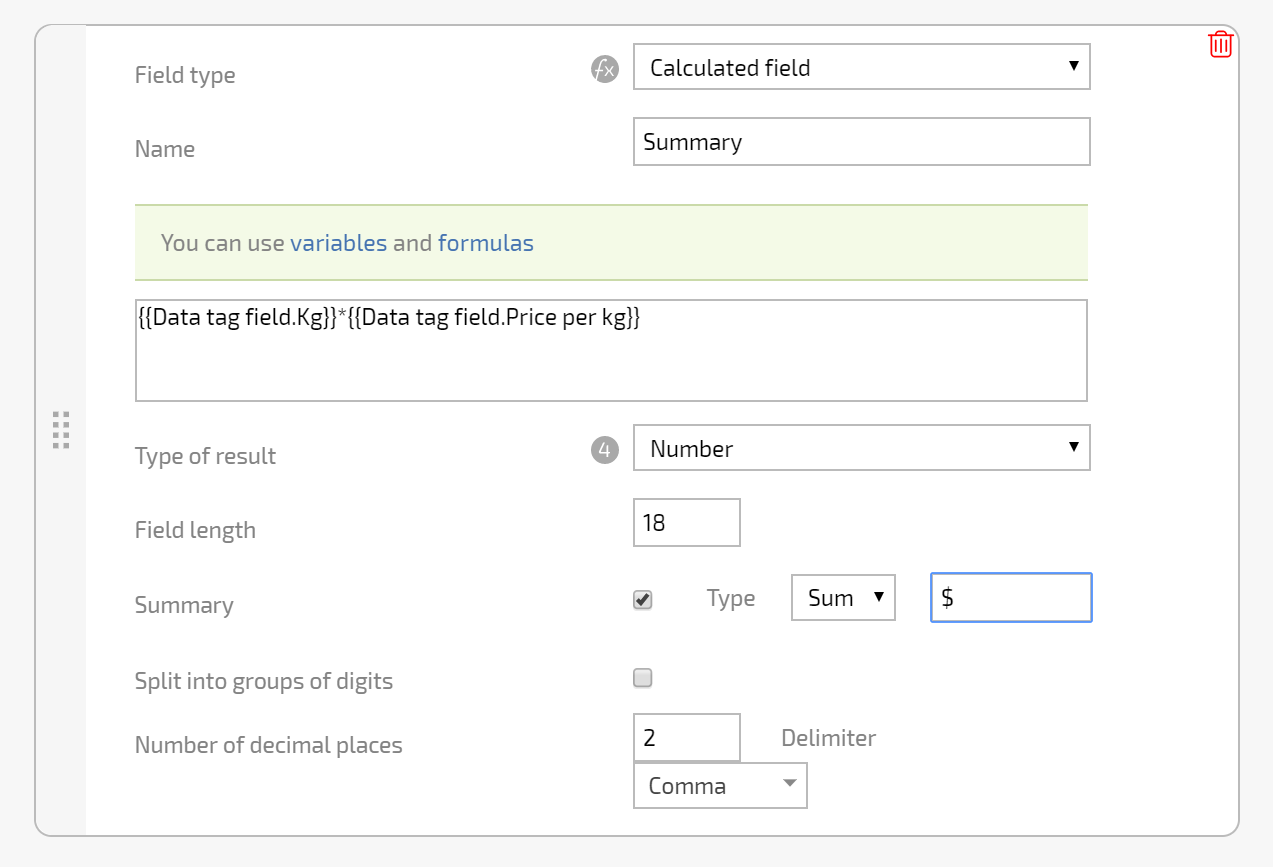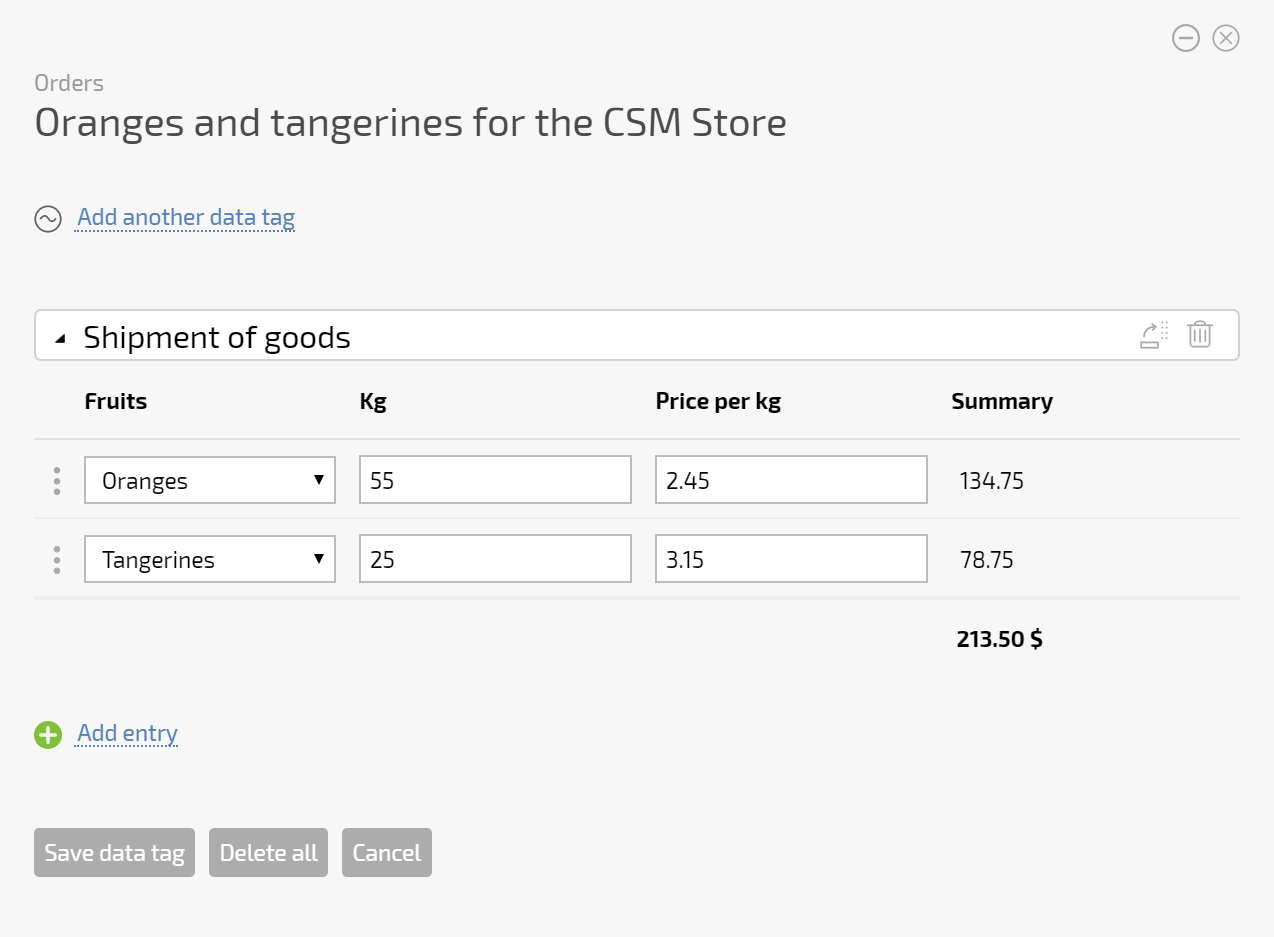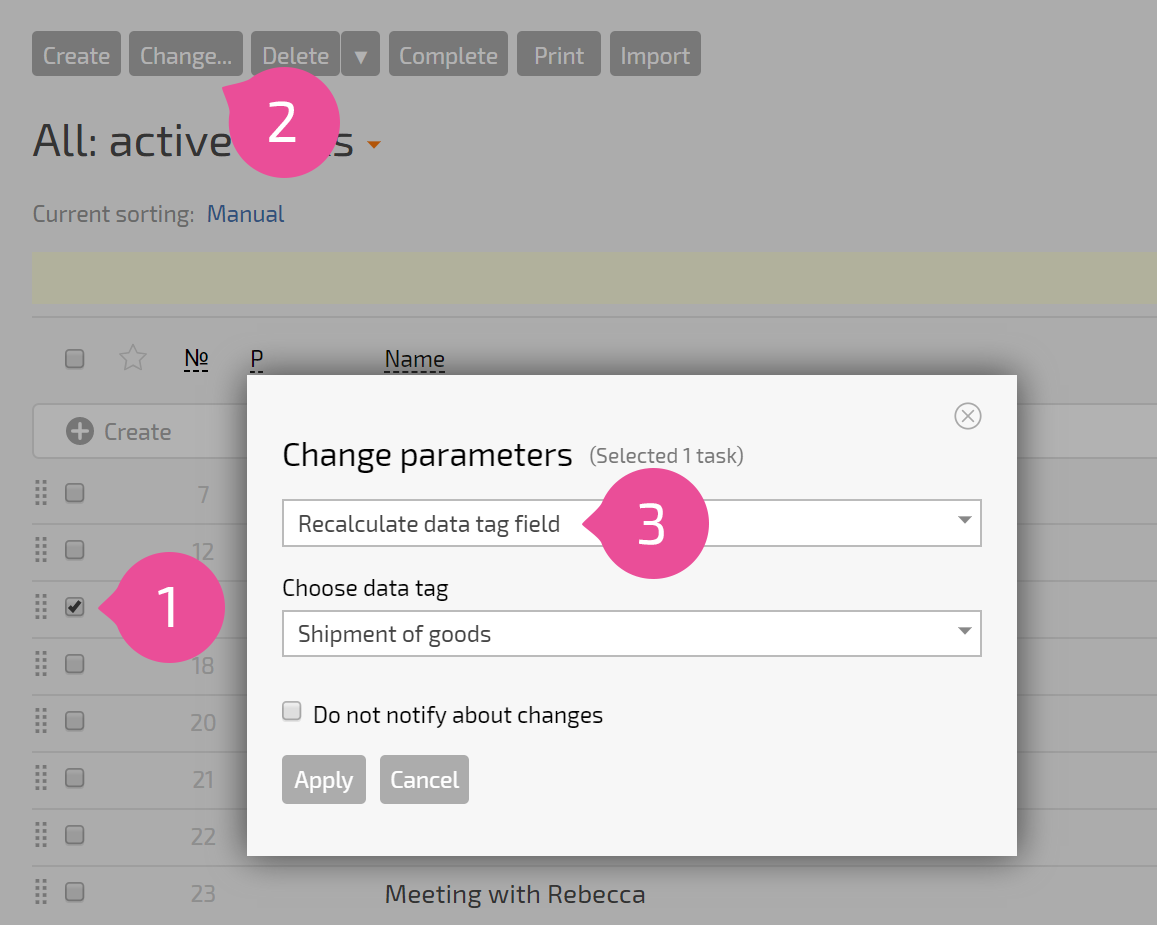# Calculated data tag fields

There is a type of Calculated Field available in data tags. These calculated fields use fields from the data tags where they're added.

To use this type of calculated field, add the following type of field to the data tag:Sample field settings:Any standard Planfix function can be used in a calculated field formula. The value of the calculated field is automatically recalculated when you enter data in fields that are part of the formula.If you change the value of any of the fields that are part of the formula, the calculated field is automatically recalculated.

Please note: Calculated field values in previously entered data tags can be manually recalculated. To do this, select the necessary tasks or contacts (or all tasks or contacts) with this data tag and apply the bulk action Recalculate data tag field: